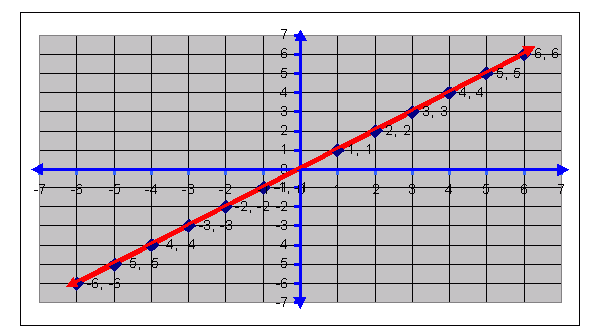Email us to get an instant 20% discount on highly effective K-12 Math & English kwizNET Programs!

#### Online Quiz (WorksheetABCD)

Questions Per Quiz = 2 4 6 8 10

### Middle/High School Algebra, Geometry, and Statistics (AGS)1.2 Graph for Straight Line y = x

 Plot the following points on a graph sheet and join then. (1,1),(2,2),(3,3),(4,4),(5,5),(6,6),(-1,-1),(-2,-2),(-3,-3),(-4,-4),(-5,-5) and (-6,-6).Observing the above graph. 1. All these points lie on a straight line. 2. The point whose x-coordinate is equal to y-coordinate lie on this straight line. 3. Every ordered pair that satisfies the relation y = x corresponds to exactly one point on the straight line. 4. The straight line is called the graph of y = x. 5. y = x is the equation of the straight line. 6. The set of ordered pairs satisfying the equation y = x is infinite.Ā The line extends infinitely on either sides.